# The Mandelbrot set

The Mandelbrot set is a famous mathematical object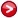defined by a very simple rule. Nevertheless the Mandelbrot set itself possesses interesting and complex properties which can be seen graphically. This is undoubtly related to the fact that it is a fractal object.

The main aim of this page is to present a small Javascript program that generates this set - see image below. Click to zoom in and shift+click to zoom out.

The rest of this page contains a brief and laid-back discussion of some basic features of this curious object.

## Generating the set

Consider a fixed complex number $c$. Starting with $z_0=0$ we can generate the sequence of numbers $z_0,z_{1},z_{2},z_{3},\cdots$ by following the rule $$z_{n+1}={z_n}^2+c$$

We may ask: is this sequence bounded (there is some limit $L$ such that $\left|z_n\right|<L$ for all $n$'s) or not ($z_n$ keeps growing indefinitely)? The $c$ number belongs to the Mandelbrot set if and only if the $z_n$ sequence is bounded.

Graphically, we may represent the numbers in this set as black dots on the complex plane. We get the following:To get an idea of the size and location of what we are talking about I have included in this picture two reference points. So this is the Mandelbrot set and its fractal nature comes from the fact that zooming in on its border region reveals smaller clones of itself. You may have noticed that this picture is not exactly the same as the one generated by the above program, which cointains small gray areas. The reason is very simple and I explain it below (see "Number of iterations").

Different parts of the set get all sorts of names. I will just mention that the Mandelbrot set is made of shapes similar to cardioidsand circles (an infinite number of both of them),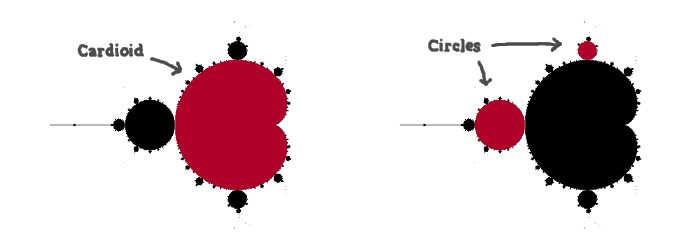So while most these are not really 'perfect circles' nor 'perfect cardioids', I will still call them circles and cardioids for simplicity. Circles are always attached to other (bigger) circles or maybe a cardioid. But the cardioids always appear as the mother 'deformed circle' to which everything else is attached.

For someone wanting to actually generate this with a small computer program, two practical questions come to mind:

• We cannot calculate the infinite sequence of $z_n$'s so how many iterations $n$ are required? 10? 100? 1000?
• We need a limit $L$ so that if any of the $z_n$ reaches $L$ we can say that the sequence is not bounded. Is there a good value for $L$?
The last question has a simple answer: if any of the $z_n$ gets a modulus bigger than 2 ($\left|z_n\right|> 2$) the sequence of $z$'s is unbounded. Things are a little trickier for the number of iterations. And the reason for this is somewhat related to the fact that the Mandelbrot set is a fractal which means that is has 'infinite detail' (we can zoom in on it forever and never get bored). So the number of iterations needed to get a sharp image of the set depends to the size of the smallest details we want to see. We shall look into this again.

## The $z_n$'s point of no return

I will assume that we've picked a $c$ and along the resulting sequence of $z$'s there is a $z_n$ such that $$\left|z_{n}\right|>2\textrm{ and }\left|z_{n}\right|>\left|c\right|$$ then $$\left|z_{n+1}\right|-\left|c\right|=\left|z_{n}^{2}+c\right|-\left|c\right|>2\left(\left|z_{n}\right|-\left|c\right|\right)$$ or $$\left|z_{n+m}\right|>\left|c\right|+2^{m}\left(\left|z_{n}\right|-\left|c\right|\right)$$ which means that the sequence is not bounded and will go to infinity.

Notice that if $\left|c\right|>2$ then $\left|z_2\right|=\left|c^2+c\right|>\left|c\right|$ which means that we get into the situation just described (with $n=2$). So the conclusions are:

• If $\left|c\right|>2$ the $z_n$ sequence is unbounded so the whole Mandelbrot set is within a circle of radius 2 centered on the origin of the complex plane;
• Even for $\left|c\right|\leq2$, if we find a $z_i$ such that $\left|z_i\right|>2$ then $c$ does not belong to the Mandelbrot set.

## Number of iterations ($n_{\textrm{max}}$)

Here is what happens when we use 5, 10, 20, 50, 100 and 2000 iterations:We get a more acurate picture of the Mandelbrot set as we increase the number of iterations. But still, it is every interesting to know how many iterations does it take us to find that a point is not in the set. To put it another way, it is interesting to know how close a point was to being included in the set.

A simple way to put this information in an image is to use a grayscale color scheme instead of the basic black & white one. For each point, we compute the number of iterations $n$ needed for the $z$'s sequence to go behond the limit $L=2$ and we color it acording to the ratio $n/n_{\textrm{max}}$. So the Mandelbrot set itself is still kept black (since in this case $n=n_{\textrm{max}}$) but points outside the set are made lighter or darker depending on the how fast the $z$'s sequence gets outside the circle of radius 2 centered on the origin of the complex plane.

To get an idea of this procedure, here is a rough version of it: with the six pictures above if we give lighter tones to the ones with less iterations and then stack them all on top of each other we get the following: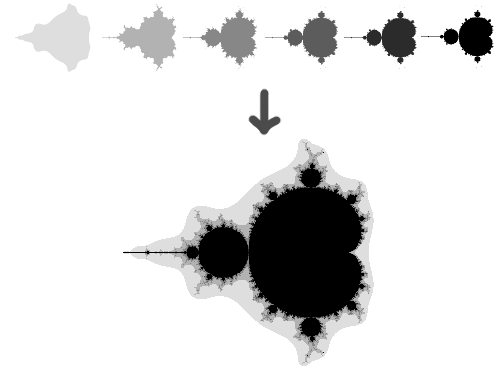## Introducing color

Instead of black & white or grayscale pictures, we can obviously arrange some other scheme to bring colors to these graphics. I chose to implement one such scheme (click "Options" above to pick it) that actually shows another interesting feature of the Mandelbrot set. It works as follows: a point $c$ in the set is given a hue equal to $\arg (z_{n_{\textrm{max}}})=\arctan\left(\textrm{Im}z_{n_{\textrm{max}}}/\textrm{Re}z_{n_{\textrm{max}}}\right)$ (scaled appropriatly so that we end up with a number in the range 0 - 255). The number $z_{n_{\textrm{max}}}$ is the last one calculated in the $z$'s sequence. We get something like this: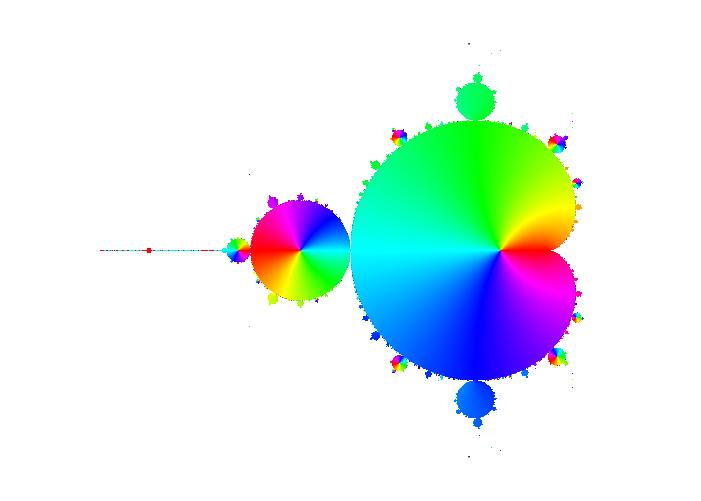To me, a non-expert, this seemed at first very strange. We see clearly that some circles appear different from the others: most circles seem to borrow the color from the cardioid/circle to which they are attached. But there are some circles which do not follow this rule and instead take on all the colors available. So is it really true that among the circles there are some special ones? No, it isn't and to see why it is worth noting that this figure was obtained using 500 (maximum) iterations. If we use, say, 600 we get this: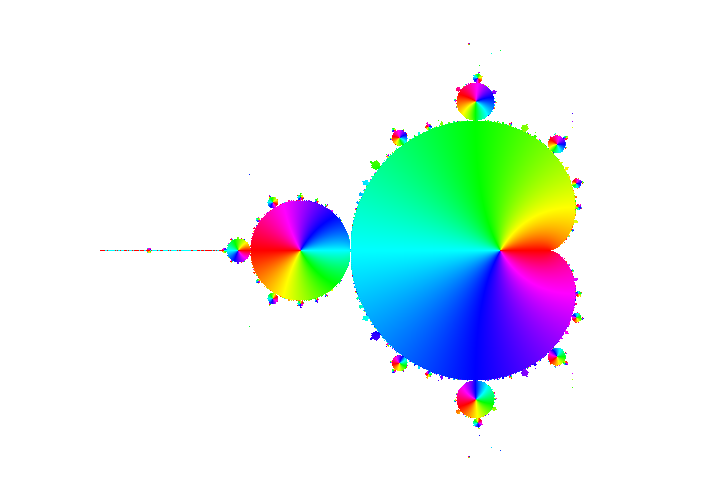The circles which appear 'special' are now different ones. So why do some circles appear with the rainbow effect? According to the chosen coloring scheme, if the hue changes quickly around a central point it means that $\arg (z_{n_{\textrm{max}}})$ is changing fast. If we consider that $z_{n_{\textrm{max}}}$ changes smoothly with the initial $c$ value then this seems to point to the fact that the 'special' circles contain a central $c$ point for which $z_{n_{\textrm{max}}}=0$. This would explain the fast changing of $\arg (z_{n_{\textrm{max}}})$ round it.

This hypothesis is indeed correct: each circle contains a central point for which there is a minimal integer $p$ (the period) such that $z_p=0$. Obviously for any multiple $np$ of $p$ we also get $z_{np}=0$. The same is true for the 'mother' circles - the cardioids. I have done a little bit of searching and catalogued the position of all the circles/cardioids with periods up to 5:Some of the cardioids are too small to be seen in the picture so the arrows seem to point to empty space. But they are there - this can be checked with the Javascript program at the top of this page.

Another way to look at this is to simply find the solutions of the equation $z_p=0$ for some period $p$. Even for small values of $p$ this can be a problem since the depence of the $z_n$'s on the seed $c$ quickly becomes a big polynomial:

\begin{eqnarray*} z_{0} & = & 0\\ z_{1} & = & c\\ z_{2} & = & c+c^{2}\\ z_{3} & = & c+c^{2}+2c^{3}+c^{4}\\ z_{4} & = & c+c^{2}+2c^{3}+5c^{4}+6c^{5}+6c^{6}+4c^{7}+c^{8}\\ z_{5} & = & c+c^{2}+2c^{3}+5c^{4}+14c^{5}+26c^{6}+44c^{7}+69c^{8}+94c^{9}+114c^{10}+116c^{11}+94c^{12}+60c^{13}+28c^{14}\\ & & +8c^{15}+c^{16}\\ & \cdots \end{eqnarray*}

I will not write down here the expression for $z_{10}$ since it would require 34164 characters (!) to do so but I have numerically solved the equation $z_{10}=0$ and the position of the solutions in the complex plane is the following: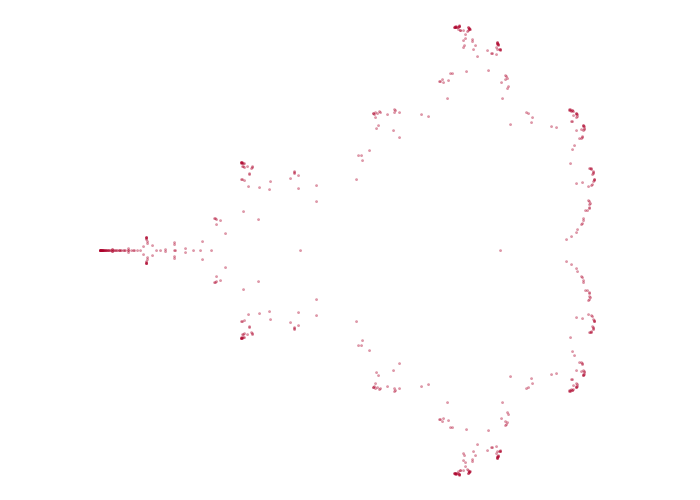Note that $z_n$ is a polynomial of degree 512 (in general $z_n$ is a polynomial fuction of $c$ of degree $2^{n-1}$) and we do get 512 distict solutions which suggests that all the roots of $z_n$ are simple.

## Other things

There is for sure a lot more to be said about the Mandelbrot set. For example, other interesting sets are obtained by changing the quadratic relation $z_{n+1}=z^2_n+c$. Another possibility is to use $z_{0}=k$ with $k\neq0$ - see Julia set here.

Even $\pi=3.1415926535\cdots$ is known to show up in some limits. For instance, near $c=-0.75$ and $c=0.25$ the number of iterations $n$ needed until $\left|z_{n}\right|\geq2$ is as follows.

 $\boldsymbol{c}$ $\boldsymbol{n}$ $-0.75+1\,i$ 3 $-0.75+0.1\,i$ 33 $-0.75+0.01\,i$ 315 $-0.75+0.001\,i$ 3143 $-0.75+0.0001\,i$ 31417 $-0.75+0.00001\,i$ 314160 $-0.75+0.000001\,i$ 3141593 $-0.75+0.0000001\,i$ 31415927 $-0.75+0.00000001\,i$ 314159266
 $\boldsymbol{c}$ $\boldsymbol{n}$ $0.25+1\,i$ 2 $0.25+0.01\,i$ 30 $0.25+0.0001\,i$ 312 $0.25+0.000001\,i$ 3140 $0.25+0.00000001\,i$ 31414 $0.25+0.0000000001\,i$ 314157 $0.25+0.000000000001\,i$ 3141591 $0.25+0.00000000000001\,i$ 31415925 $0.25+0.0000000000000001\,i$ 314159263Author
Renato Fonseca

Emails
renatofonseca@gmail.com
or
fonseca@ipnp.mff.cuni.cz

Last updated
08 April 2020